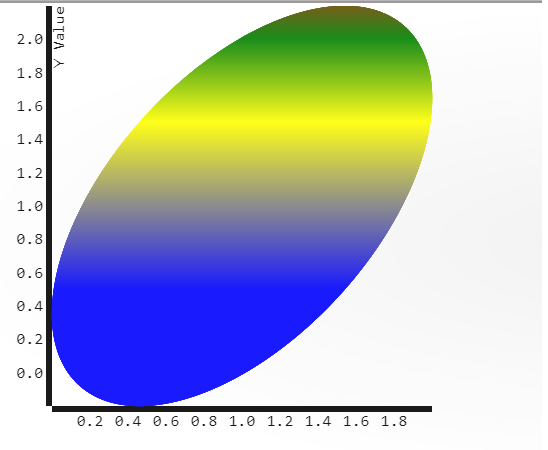# Manuals/calci/LISSAJOUSCURVE

LISSAJOUSCURVE (Type,Div,Settings)

•is the Type of the curve.

## Description

• This function shows the Lissajous curve for each values.
• Lissajous Curve is a parametric plot of the harmonic system.
• It is also called Bowditch Curves.Lissajous used sounds of different frequencies to vibrate a mirror.
• A beam of light reflected from the mirror, was allowed to trace patterns which depended on the frequencies of the sounds – in a setup similar to projectors used in today's laser light shows.
• Lissajous figure is the intersection of two sinusoidal curves, the axes of which are at right angles to each other.
• Mathematically, this translates to a Complex harmonic function:,• The appearance of a figure is highly sensitive to a/b, the ratio of a and b.
• According to the ratio value, the shapes of the figures change in interesting ways.
• For a a/b ratio=1, the figure is an ellipse.
• For a=b,=radians, the figure is a circle.
• For= 0, the figure is a line.
• For a/b = 2,=, the result is a parabola.
• The Lissajous curve gets more complicated for other ratios, which are closed only if a/b is rational.

## Examples

1. LISSAJOUSCURVE("ellipse")Lissajous